### Playing with Numbers-Solutions Ex. 3.5

CBSE Class –VI Mathematics
NCERT Solutions
Chapter 3 Playing With Numbers (Ex. 3.5)

Question 1. Which of the following statements are true?
(a) If a number is divisible by 3, it must be divisible by 9.
(b) If a number is divisible by 9, it must be divisible by 3.
(c) If a number is divisible by 18, it must be divisible by both 3 and 6.
(d) If a number is divisible by 9 and 10 both, then it must be divisible by 90.
(e) If two numbers are co-prime, at least one of them must be prime.
(f) All numbers which are divisible by 4 must also be divisible by 8.
(g) All numbers which are divisible by 8 must also be divisible by 4.
(h) If a number is exactly divides two numbers separately, it must exactly divide their sum.
(i) If a number is exactly divides the sum of two numbers, it must exactly divide the two numbers separately.
Answer: Statements (b), (c), (d), (g) and (h) are true.
Question 2. Here are two different factor trees for 60. Write the missing numbers.
(a)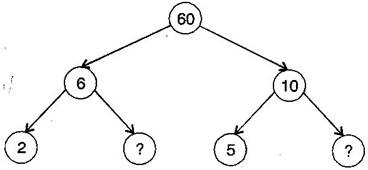(b)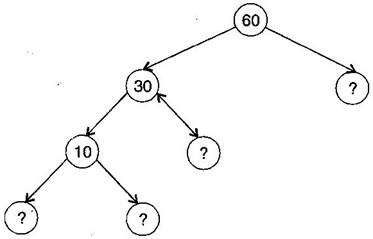(a)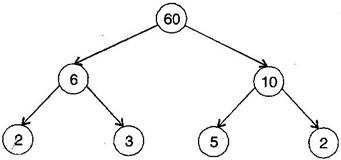(b)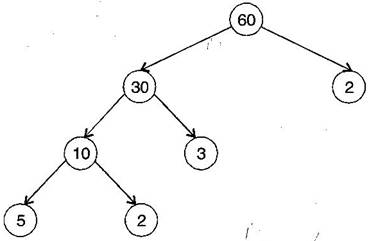Question 3. Which factors are not included in the prime factorization of a composite number?
1 is not included in the prime factorization of a composite number.
Question 4. Write the greatest 4-digit number and express it in terms of its prime factors.
Answer: The greatest 4-digit number is 9999.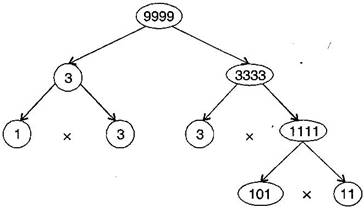The prime factors of 9999 are 3 x 3 x 11 x 101.
Question 5. Write the smallest 5-digit number and express it in terms of its prime factors.
Answer: The smallest 5-digit number is 10000.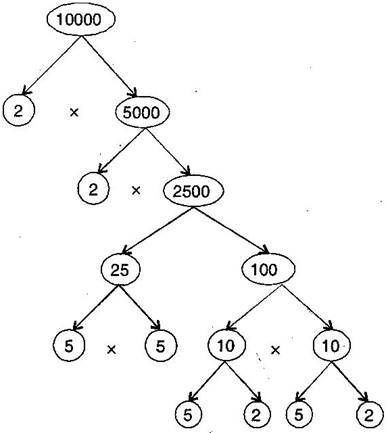The prime factors of 10000 are 2 x 2 x 2 x 2 x 5 x 5 x 5 x 5.

Question 6. Find all the prime factors of 1729 and arrange them in ascending order. Now state the relation, if any, between, two consecutive prime numbers.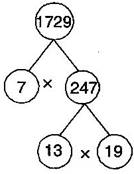Prime factors of 1729 are 7 x 13 x 19.
The difference of two consecutive prime factors is 6.
Question 7. The product of three consecutive numbers is always divisible by 6. Verify this statement with the help of some examples.
Answer: Among the three consecutive numbers, there must be one even number and one multiple of 3. Thus, the product must be multiple of 6.
Example:
(i) 2 x 3 x 4 = 24
(ii) 4 x 5 x 6 = 120
Question 8. The sum of two consecutive odd numbers is always divisible by 4. Verify this statement with the help of some examples.
Answer: 3 + 5 = 8 and 8 is divisible by 4.
5 + 7 = 12 and 12 is divisible by 4.
7 + 9 = 16 and 16 is divisible by 4.
9 + 11 = 20 and 20 is divisible by 4.
Question 9. In which of the following expressions, prime factorization has been done:
(a) 24 = 2 x 3 x 4
(b) 56 = 7 x 2 x 2 x 2
(c) 70 = 2 x 5 x 7
(d) 54 = 2 x 3 x 9
Answer: In expressions (b) and (c), prime factorization has been done.
Question 10. Determine if 25110 is divisible by 45.
[Hint: 5 and 9 are co-prime numbers. Test the divisibility of the number by 5 and 9.]
Answer: The prime factorization of 45 = 5 x 9
25110 is divisible by 5 as ‘0’ is at its unit place.
25110 is divisible by 9 as sum of digits is divisible by 9.
Therefore, the number must be divisible by 5 x 9 = 45
Question 11. 18 is divisible by both 2 and 3. It is also divisible by 2 x 3 = 6. Similarly, a number is divisible by 4 and 6. Can we say that the number must be divisible by 4 x 6 = 24? If not, give an example to justify your answer.
Answer: No. Number 12 is divisible by both 6 and 4 but 12 is not divisible by 24.
Question 12. I am the smallest number, having four different prime factors. Can you find me?
Answer: 2 x 3 x 5 x 7 = 210